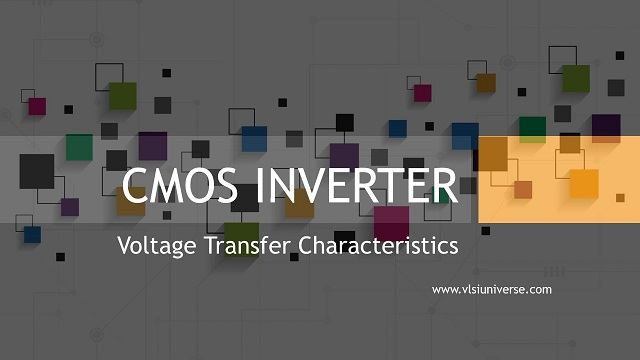You are here
Home > CMOS >

# CMOS Inverter | VTC | Noise Margin June 2021One of the main advantages of the CMOS inverter is it consumes power only during the transients/operation. At the steady-state, it consumes no power.

The difference between hole mobility and electron mobility can be compensated and make tphl and tplh, rise, and fall delays more compatible by designing or adjusting the width by length W/L ratios of the PMOS and NMOS devices. The symmetric tphl and tplh, rise and fall delays facilitate the very easy circuit design.

In CMOS inverter the input-output I/O transfer curve can be symmetric wrt. (with respect to) the center of the signal swing so that the NM noise margin can be optimized here.

The load capacitance CL can be reduced by scaling. And by increasing the width by length W/L ratios or aspect ratio, the parasitic capacitance at the output may increase, which will not reduce the tp, the propagation delay.

This also may lead to an increase in the power consumption of the circuit. And beta n and beta p can be increased by decreasing the gate oxide thickness tox and increasing the W/L, the aspect ratio.The static CMOS inverter is shown above with input voltage Vin and output Vout, The terminal points G, S, and D indicate gate, source, and drain terminals of the PMOS(load) and NMOS(driver) transistors respectively.The above figure shows the voltage transfer characteristics of the CMOS inverter. The VTC of the CMOS inverter can be divided into five different regions to understand its operation.

Those are based on the gate to source voltage Vgs that is input to the inverter. region 1 Vgs is from 0v to Vtn, region 2 Vgs is from Vtn to Vdd/2, region 3 Vgs is from Vdd/2, region 4 Vgs is from Vdd/2 to Vdd-|Vtp|, region 5 Vgs is from Vdd-|Vtp| to Vdd.

### The operation of CMOS inverter

 Regions of operation When Vin is or is between Status of PMOS transistor (load) Status of NMOS transistor (driver) A)0 to Vtn Linear region Cut-off region B)Vtn to Vdd/2 Linear region Saturation region C)Vdd/2 Saturation region Saturation region D)Vdd/2 to Vdd-|Vtp| Saturation region Linear region E)Vdd-|Vtp| to Vdd Cut-off region Linear region

1. The operation of the inverter can be divided into five and the status of the transistor at each region is shown above in the table.

2. For the ideal transistors (region C operation for Vin = Vdd/2), the slope of the voltage transfer curve will infinite gain(-infinity). And for the real transistors, the slope of the voltage transfer curve VTC will have a finite gain because of the channel length modulation CLM and the output resistances over a broader region in region C.

3.Ideally, the CMOS inverters consume the Zero current, while neglecting the leakage, when the input is within the threshold voltage of the supply Vdd or ground GND rails.

The point where the DC load line when Vin = Vout intersects with the voltage transfer curve VTC called input threshold point. At this point, the mobility and the value of threshold voltage Vth for both NMOS and PMOS transistors decrease with temperature. That means the input threshold becomes weakly sensitive to temperature.

The relation for input threshold voltage is given byThe current equations at different regions of operations are given by

Ids = 0 ; Vgs < Vth ; The cut-off region

Ids = β (Vgs – Vth – Vdd/2) ; Vds </= Overdrive voltage(Vgs – Vth) ; The linear region

Ids = β (Vgs – Vth)^2 ; Vds > Overdrive voltage (Vgs – Vth) ; The saturation region.